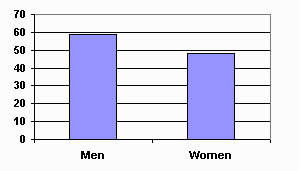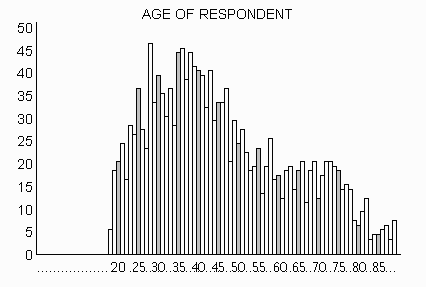Measures of Central Tendency:
Mean, Median, & Mode

All files, sofware, and tutorials that make up SABLE Copyright (c) 1997 1998 1999 Virginia Tech. You may use these programs under the conditions of the SABLE General License, which incorporates the GNU GENERAL PUBLIC LICENSE.

## Introduction

This tutorial uses histograms to illustrate different measures of central tendency. A histogram is a type of graph in which the x-axis lists categories or values for a data set, and the y-axis shows a count of the number of cases falling into each category. For example, if there are 59 men and 48 women in your class, you could represent the information with this histogram:The categories may be non-numeric, as in the histogram above, or may be numeric, as in the following histogram. The x-axis shows the ages for respondents to a survey and the y-axis reports the frequency or count for occurrances of each age.From the histogram, can you determine what is the "typical" age of the participants in the survey?   This question could be answered in several different ways, depending on what you really want to know. Do you want to determine:

• The average of the ages?
• The age which divides the cases into two equal-sized groups -- the "highs" vs. the "lows"?
• The most common age?
Questions like these are concerned with determining the central tendency of a group of numbers or data. To answer our question, we want a single number which can somehow represent all of the ages of the people who participated in the survey.

## Ways to Measure Central Tendency

The three most commonly-used measures of central tendency are the following.
mean
The sum of the values divided by the number of values--often called the "average."
• Add all of the values together.
• Divide by the number of values to obtain the mean.
Example: The mean of 7, 12, 24, 20, 19 is (7 + 12 + 24 + 20 + 19) / 5 = 16.4.
median
The value which divides the values into two equal halves, with half of the values being lower than the median and half higher than the median.
• Sort the values into ascending order.
• If you have an odd number of values, the median is the middle value.
• If you have an even number of values, the median is the arithmetic mean (see above) of the two middle values.
Example: The median of the same five numbers (7, 12, 24, 20, 19) is 19.
mode
The most frequently-occurring value (or values).
• Calculate the frequencies for all of the values in the data.
• The mode is the value (or values) with the highest frequency.
Example: For individuals having the following ages -- 18, 18, 19, 20, 20, 20, 21, and 23, the mode is 20.
Check your understanding of these concepts by calculating the mean, median, and mode of the following three sets of numbers.

## Which Measure Should You Use?

This histogram shows the distribution of the number of siblings for survey respondents. The mode (i.e., most common number of siblings) is easy to find.  Can you also determine the median simply by inspection?   What about the mean?

You should see two copies of the histogram. The upper histogram allows you to drag the red vertical line to help locate the median. Numbers on either side of the red line show you how many values exist above and below the line.

The lower histogram allows you to move a triangle within the range of the distribution which acts like a fulcrum for a see-saw. The mean is located at the point where the histogram is balanced. Use these tools -- the red vertical line and the fulcrum -- to find the median and mean of the data.

Now write down which of these three measures of central tendency (mean, median, or mode) you think best describes the "typical" number of siblings of the respondents. Explain why you chose the one you did.

You can use the histogram activity to explore other variables from the the 1993 General Social Survey. The available variables appear under the "Dataset" menu in the histogram window. Look at several of the variables, and use the tools to find  the mean and median for each one.

Notice that not all measures of central tendency are appropriate for all kinds of variables. For example,

• For nominal data (such as sex or race), the mode is the only valid measure.
• For ordinal data (such as salary categories), only the mode and median can be used.
Now explain in your own words how the three measures of central tendency differ from one another. In the space below, briefly answer the following three questions:
1. Why is the mean not appropriate for some types of data?
2. When do you want to use the median rather than the mean?
3. When would the mode be most appropriate?

Use the button to . Now compare your responses with the guidelines given in "Choosing an Appropriate Measure of Central Tendency."

## Skewness

In a normal distribution, the mean, median, and mode are all the same value. In various other symmetrical distributions it is possible for the mean and median to be the same even though there may be several modes, none of which is at the mean. By contrast, in asymmetrical distributions the mean and median are not the same. Such distributions are said to be skewed, i.e., more than half the cases are either above or below the mean.

Below are some exercises that illustrate the relationship between mean, median, and mode in skewed distributions. In each exercise you will be asked to modify a histogram that satisfies certain conditions. You can change each histogram by dragging the mouse across it with the button down. You can then check your answer by clicking the ``Done'' button.

At this point, you should have created a symmetrical distribution, a negatively skewed distribution, and a positively skewed distribution. If you think about the three figures, you can deduce a general rule about the relationship between the symmetry of a distribution of scores and measures of central tendency. The rule is that, as the symmetry of a distribution increases, the three measures of central tendency converge on the same value. As the asymmetry or skewness of a distribution increases, the three measures of central tendency diverge systematically.

For a positively skewed distribution, the mean will always be the highest estimate of central tendency and the mode will always be the lowest estimate of central tendency (assuming that the distribution has only one mode). For negatively skewed distributions, the mean will always be the lowest estimate of central tendency and the mode will be the highest estimate of central tendency.

In any skewed distribution (i.e., positive or negative) the median will always fall in-between the mean and the mode. As previously discussed in the section on "choosing an appropriate measure of central tendency", when dealing with skewed distributions, researchers typically decide between the mean or median as the best estimate of central tendency. As distributions go from symmetrical to more skewed, the researcher is more likely to chose the median over the mean.

Now you should be able to look at real data sets and spot the three Measures of Central Tendency. Use this activity to examine different variables.